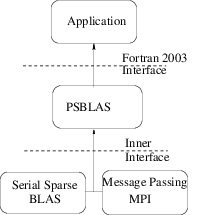### 2 General overview

The PSBLAS library is designed to handle the implementation of iterative solvers for sparse linear systems on distributed memory parallel computers. The system coefficient matrix A must be square; it may be real or complex, nonsymmetric, and its sparsity pattern needs not to be symmetric. The serial computation parts are based on the serial sparse BLAS, so that any extension made to the data structures of the serial kernels is available to the parallel version. The overall design and parallelization strategy have been influenced by the structure of the ScaLAPACK parallel library. The layered structure of the PSBLAS library is shown in figure 1; lower layers of the library indicate an encapsulation relationship with upper layers. The ongoing discussion focuses on the Fortran 2003 layer immediately below the application layer. The serial parts of the computation on each process are executed through calls to the serial sparse BLAS subroutines. In a similar way, the inter-process message exchanges are encapsulated in an applicaiton layer that has been strongly inspired by the Basic Linear Algebra Communication Subroutines (BLACS) library . Usually there is no need to deal directly with MPI; however, in some cases, MPI routines are used directly to improve efficiency. For further details on our communication layer see Sec. 7.Figure 1: PSBLAS library components hierarchy.

The type of linear system matrices that we address typically arise in the numerical solution of PDEs; in such a context, it is necessary to pay special attention to the structure of the problem from which the application originates. The nonzero pattern of a matrix arising from the discretization of a PDE is influenced by various factors, such as the shape of the domain, the discretization strategy, and the equation/unknown ordering. The matrix itself can be interpreted as the adjacency matrix of the graph associated with the discretization mesh.

The distribution of the coefficient matrix for the linear system is based on the “owner computes” rule: the variable associated to each mesh point is assigned to a process that will own the corresponding row in the coefficient matrix and will carry out all related computations. This allocation strategy is equivalent to a partition of the discretization mesh into sub-domains. Our library supports any distribution that keeps together the coefficients of each matrix row; there are no other constraints on the variable assignment. This choice is consistent with simple data distributions such as CYCLIC(N) and BLOCK, as well as completely arbitrary assignments of equation indices to processes. In particular it is consistent with the usage of graph partitioning tools commonly available in the literature, e.g. METIS . Dense vectors conform to sparse matrices, that is, the entries of a vector follow the same distribution of the matrix rows.

We assume that the sparse matrix is built in parallel, where each process generates its own portion. We never require that the entire matrix be available on a single node. However, it is possible to hold the entire matrix in one process and distribute it explicitly1 , even though the resulting memory bottleneck would make this option unattractive in most cases.